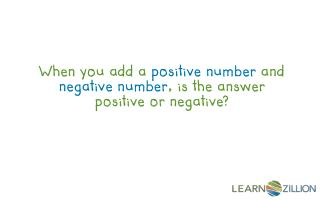# When you add a positive number and negative number , is the answer positive or negative? - PowerPoint PPT PresentationDownload PresentationWhen you add a positive number and negative number , is the answer positive or negative?

When you add a positive number and negative number , is the answer positive or negative?
Download Presentation## When you add a positive number and negative number , is the answer positive or negative?

- - - - - - - - - - - - - - - - - - - - - - - - - - - E N D - - - - - - - - - - - - - - - - - - - - - - - - - - -
##### Presentation Transcript

1. When you add a positive number and negative number, is the answer positive or negative?

2. In this lesson you will learn to add positive and negative integers by using a number line.

3. What’s an integer? Integers include positive and negative whole numbers as well as 0. ...-3, -2, -1, 0, 1, 2, 3 ... More Examples: -5 3 28 -9 1 0

5. Adding a positive and negative integer like two positives and applying the sign of the number with the larger absolute value. -4 + 6 = 10 - 4 + (-6) = -10 -

6. Positive Direction Negative Direction Negative Numbers Positive Numbers

7. 8 + (-2) =6 Negative Direction -2 8 6 Positive Numbers

8. 8 + (-13) =-5 Negative Direction -13 8 -5 Positive Numbers

9. -2 8 + (-2) =6 8 6 -13 8 + (-13)=-5 8 -5

10. In this lesson you learned to add positive and negative integers by using a number line.

11. -12 + 8 -12 + 15

12. What is the sum of 14 and -5?

13. Try graphing two addition problems with opposite numbers on the same graph. • For example, use -15 + 3 and 15 + (-3). • Write about what you notice.

14. Use what you know about adding positive and negative numbers to fill out the table using mental math.

15. Partner Game: • Write the integers -20 through +20 on small pieces of paper and turn them face down. • You and your partner pull 2 numbers. Sum the integers by graphing the process on a number line. The person with the greater sum gets a point. Repeat.

16. Simplify 9 + (-7). What is the sum of -6 and -13?